A scatter plot extension for Jupyter Notebook and Lab

# jupyter-scatter

An interactive scatter plot widget for Jupyter Notebook, Lab, and Google Colab
that can handle millions of points and supports view linking.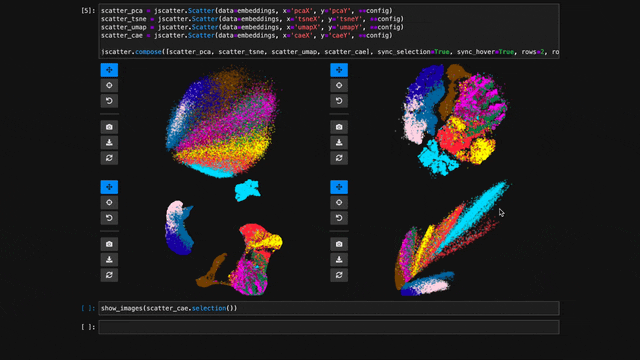Why? Imagine trying to explore an embedding space of millions of data points. Besides plotting the space as a 2D scatter, the exploration typically involves three things: First, we want to interactively adjust the view (e.g., via panning & zooming) and the visual point encoding (e.g., the point color, opacity, or size). Second, we want to be able to select/highlight points. And third, we want to compare multiple embeddings (e.g., via animation, color, or point connections). The goal of jupyter-scatter is to support all three requirements and scale to millions of points.

How? Internally, jupyter-scatter uses regl-scatterplot for rendering and ipywidgets for linking the scatter plot to the iPython kernel.

## Install

pip install jupyter-scatter


If you are using JupyterLab <=2:

jupyter labextension install @jupyter-widgets/jupyterlab-manager jupyter-scatter


For a minimal working example, take a look at test-environments.

## Get Started

To play with the following examples yourself, open notebooks/get-started.ipynb.

Note

Also check out our full-blown tutorial that we first presented at the SciPy '23 conference.

#### Simplest Example

In the simplest case, you can pass the x/y coordinates to the plot function as follows:

import jscatter
import numpy as np

x = np.random.rand(500)
y = np.random.rand(500)

jscatter.plot(x, y)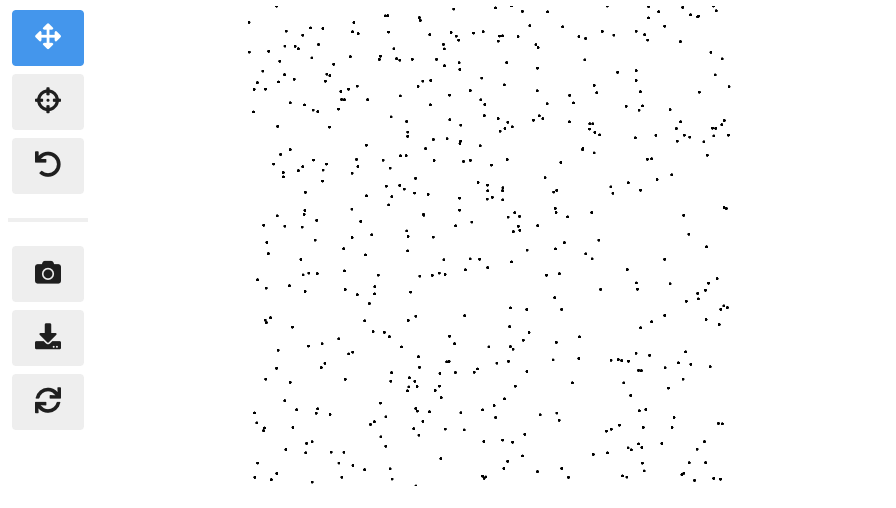#### Pandas Example

Say your data is stored in a Pandas dataframe like the following:

import pandas as pd

# Just some random float and int values
data = np.random.rand(500, 4)
df = pd.DataFrame(data, columns=['mass', 'speed', 'pval', 'group'])
# We'll convert the group column to strings to ensure it's recognized as
# categorical data. This will come in handy in the advanced example.
df['group'] = df['group'].map(lambda c: chr(65 + round(c)), na_action=None)

x y value group
0 0.13 0.27 0.51 G
1 0.87 0.93 0.80 B
2 0.10 0.25 0.25 F
3 0.03 0.90 0.01 G
4 0.19 0.78 0.65 D

You can then visualize this data by referencing column names:

jscatter.plot(data=df, x='mass', y='speed')

Show the resulting scatter plot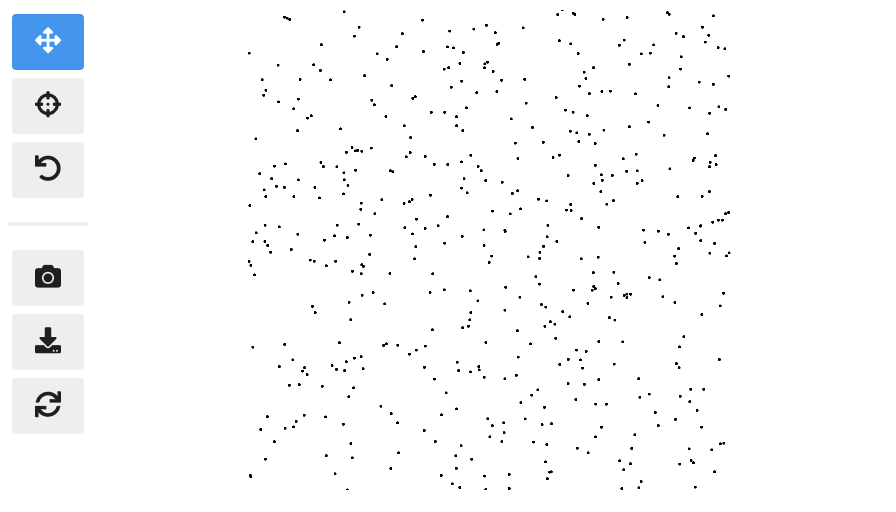Often you want to customize the visual encoding, such as the point color, size, and opacity.

jscatter.plot(
data=df,
x='mass',
y='speed',
size=8, # static encoding
color_by='group', # data-driven encoding
opacity_by='density', # view-driven encoding
)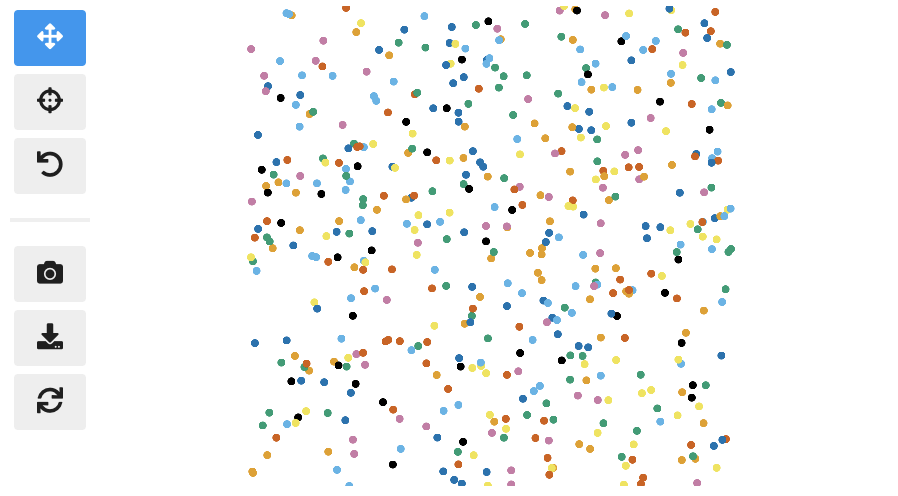In the above example, we chose a static point size of 8. In contrast, the point color is data-driven and assigned based on the categorical group value. The point opacity is view-driven and defined dynamically by the number of points currently visible in the view.

Also notice how jscatter uses an appropriate color map by default based on the data type used for color encoding. In this examples, jscatter uses the color blindness safe color map from Okabe and Ito as the data type is categorical and the number of categories is less than 9.

Important: in order for jscatter to recognize categorical data, the dtype of the corresponding column needs to be category!

You can, of course, customize the color map and many other parameters of the visual encoding as shown next.

#### Functional API Example

The flat API can get overwhelming when you want to customize a lot of properties. Therefore, jscatter provides a functional API that groups properties by type and exposes them via meaningfully-named methods.

scatter = jscatter.Scatter(data=df, x='mass', y='speed')
scatter.selection(df.query('mass < 0.5').index)
scatter.color(by='mass', map='plasma', order='reverse')
scatter.opacity(by='density')
scatter.size(by='pval', map=[2, 4, 6, 8, 10])
scatter.height(480)
scatter.background('black')
scatter.show()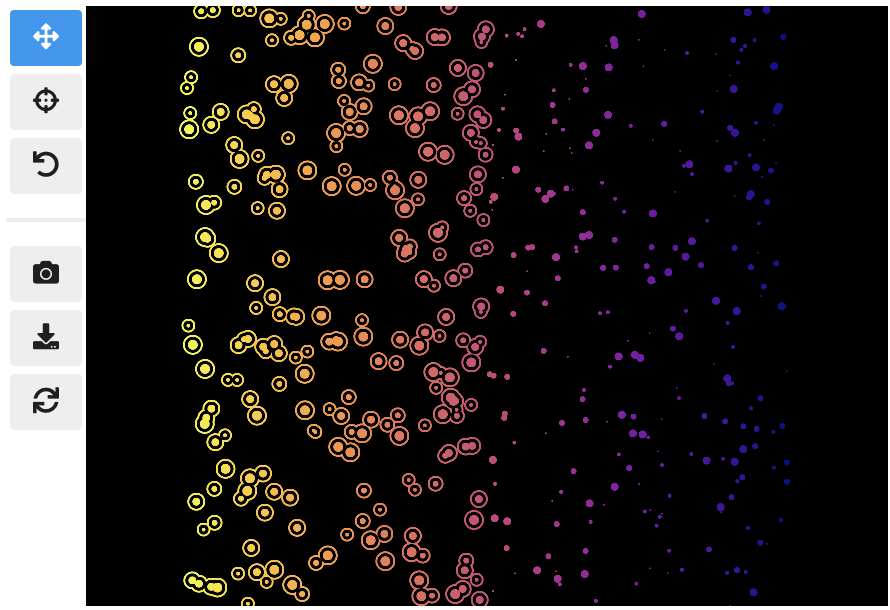When you update properties dynamically, i.e., after having called scatter.show(), the plot will update automatically. For instance, try calling scatter.xy('speed', 'mass')and you will see how the points are mirrored along the diagonal.

Moreover, all arguments are optional. If you specify arguments, the methods will act as setters and change the properties. If you call a method without any arguments it will act as a getter and return the property (or properties). For example, scatter.selection() will return the currently selected points.

Finally, the scatter plot is interactive and supports two-way communication. Hence, if you select some point with the lasso tool and then call scatter.selection() you will get the current selection.

To explore multiple scatter plots and have their view, selection, and hover interactions link, use jscatter.link().

jscatter.link([
jscatter.Scatter(data=embeddings, x='pcaX', y='pcaY', **config),
jscatter.Scatter(data=embeddings, x='tsneX', y='tsneY', **config),
jscatter.Scatter(data=embeddings, x='umapX', y='umapY', **config),
jscatter.Scatter(data=embeddings, x='caeX', y='caeY', **config)
], rows=2)


https://user-images.githubusercontent.com/932103/162584133-85789d40-04f5-428d-b12c-7718f324fb39.mp4

#### Visualize Millions of Data Points

With jupyter-scatter you can easily visualize and interactively explore datasets with millions of points.

In the following we're visualizing 5 million points generated with the Rössler attractor.

points = np.asarray(roesslerAttractor(5000000))
jscatter.plot(points[:,0], points[:,1], height=640)


https://user-images.githubusercontent.com/932103/162586987-0b5313b0-befd-4bd1-8ef5-13332d8b15d1.mp4

See notebooks/examples.ipynb for more details.

While jscatter is primarily developed for Jupyter Lab and Notebook, it also runs just fine in Google Colab. See jupyter-scatter-colab-test.ipynb for an example.

### Development

Setting up a development environment

Requirements:

Installation:

git clone https://github.com/flekschas/jupyter-scatter/ jscatter && cd jscatter
conda env create -f environment.yml && conda activate jscatter
pip install -e ".[test]"


After Changing Python code: simply restart the kernel.

After Changing JavaScript code: do cd js && npm run build. Alternatively you can run npm run watch and rebundle the code on the fly.

Setting up a test environment

Go to test-environment and follow the detailed instructions

## Project details

This version0.14.30.14.20.14.10.14.00.13.10.13.00.12.60.12.50.12.40.12.30.12.20.12.10.12.00.11.00.10.00.9.00.8.00.7.30.7.20.7.10.7.00.6.10.6.00.5.10.5.00.4.10.4.00.3.40.3.30.3.20.3.10.3.00.2.20.2.10.2.00.1.10.1.0

Uploaded source
Uploaded py2 py3